# The Futurama Theorem

### Dana C. Ernst

Northern Arizona University
http://danaernst.com

#### DePaul Mathematics Club

September 15, 2017

## Futurama

• Futurama is an American animated science fiction sitcom created by Matt Groening (of The Simpsons).
• Aired on Fox from 1999-2013 with some time off in the middle.
• Show follows the adventures of pizza delivery boy, Philip J. Fry, who was accidentally frozen in 1999 and then thawed out on New Year's Eve 2999.
• Some main characters: Fry, Leela (one-eyed alien), Bender (robot), Professor Farnsworth (Fry's distant nephew and mad scientist), Zoidberg (lobster-like alien), Amy (spoiled and accident prone student), and more.

## Futurama

Here is short clip with Professor Farnsworth and the Globe Trotters.

## The Prisoner of Benda

• "The Prisoner of Benda" is Episode 10 of Season 6 and aired in August 2010.
• The title is a play on "The Prisoner of Zenda", a 1894 novel by Anthony Hope Hawkins.
• During episode, the Professor and Amy create a mind-switching machine but quickly discover a serious limitation: when two bodies have switched minds, they can never switch back with each other.
• After a mind-switching bonanza involving several of the show’s characters, the Professor aided by the Globetrotters try to find a way to return each mind to its rightful owner.
• Episode written by Ken Keeler, who has a PhD in applied mathematics from Harvard.
• Keeler proved a new theorem specifically for the show.

## The Futurama Mind Swap Problem

Here are the swaps that take place during the episode. We are indicating which bodies sat in machine.

• Professor ($p$) $\leftrightarrow$ Amy ($a$)
• Amy ($a$) $\leftrightarrow$ Bender ($b$)
• Professor ($p$) $\leftrightarrow$ Leela ($l$)
• Amy ($a$) $\leftrightarrow$ Wash Bucket ($w$)
• Fry ($f$) $\leftrightarrow$ Zoidberg ($z$)
• Emperor Nikolai ($e$) $\leftrightarrow$ Wash Bucket ($w$)
• Hermes ($h$)$\leftrightarrow$ Leela ($l$)

The upshot: $a \rightarrow h \rightarrow l \rightarrow p \rightarrow b \rightarrow e \rightarrow w \rightarrow a \text{ and } f \leftrightarrow z$

## The Futurama Mind Swap Problem

The question is:

Can the machine be used to put everyone's mind back in the proper body if we are not allowed to use the machine on the same pair of bodies more than once?

We need to use math!

## The Symmetric Group

We can model this problem in terms of permutations (i.e., rearrangements) of numbers. It turns out that the set of all permutations of the numbers 1 through $n$ forms a group under composition.

A group is a set with an associative binary operation satisfying:

• #### Closure

The "product" of any two elements from the set is an element of the set.
• #### Identity

There exists a "do-nothing" element.
• #### Inverses

For every element in the set, there exists another element in the set that "undoes" the original.

The group of permutations of $\{1,2,\ldots, n\}$ is called the symmetric group and is denoted by $S_n$. Note: We will multiply right to left (like function composition).

## Permutation Diagrams

One way of representing an element of the symmetric group is via permutation diagrams, which we illustrate with examples.

### Example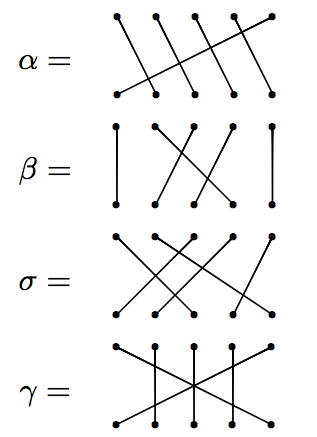## Permutation Diagrams

Let's try multiplying.

### Example

Let $\alpha$ and $\beta$ be as on previous slide. Then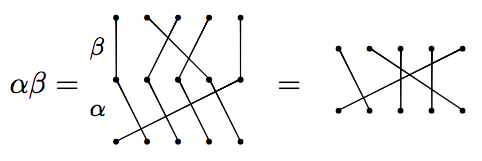But on the other hand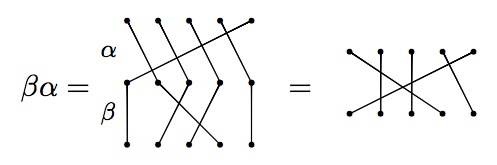We see that products of permutations do not necessarily commute (order matters).

## Permutation Diagrams

However, sometimes permutations do commute.

### Example

Let $\beta$ and $\gamma$ be as before. Then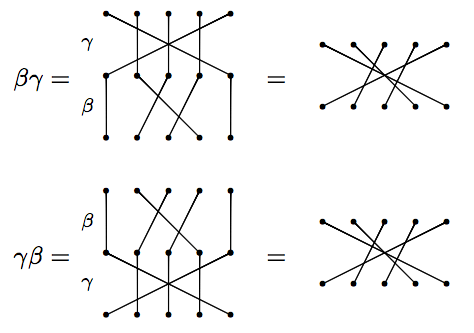Notice that these result in the same diagram. So, $\beta$ and $\gamma$ commute.

• Order matters, but not always.
• A permutation/diagram that swaps precisely two numbers is called a transposition.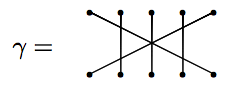• "Disjoint" transpositions commute.
• $S_n$ is generated by the transpositions. That is, every permutation can be written as a product of some sequence of transpositions. In fact, we only need the adjacent transpositions: $i$ swaps with $i+1$.

## The Futurama Mind Swap Problem as a Diagram

If we let $a=1$, $h=2$, $l=3$, $p=4$, $b=5$, $e=6$, and $w=7$, $f=8$, and $z=9$, then the mind swaps from Futurama can be depicted as:## Rephrasing the Problem

The problem can be restated as:

Suppose a permutation results from multiplying distinct transpositions. Can we multiply this permutation by a sequence of transpositions that we have not yet used to obtain the identity permutation?

It turns out that the answer is yes. Keeler's trick to pulling this off is to utilize two additional people that have not sat in the machine.

## The Futurama Theorem

Here is the solution presented in the show.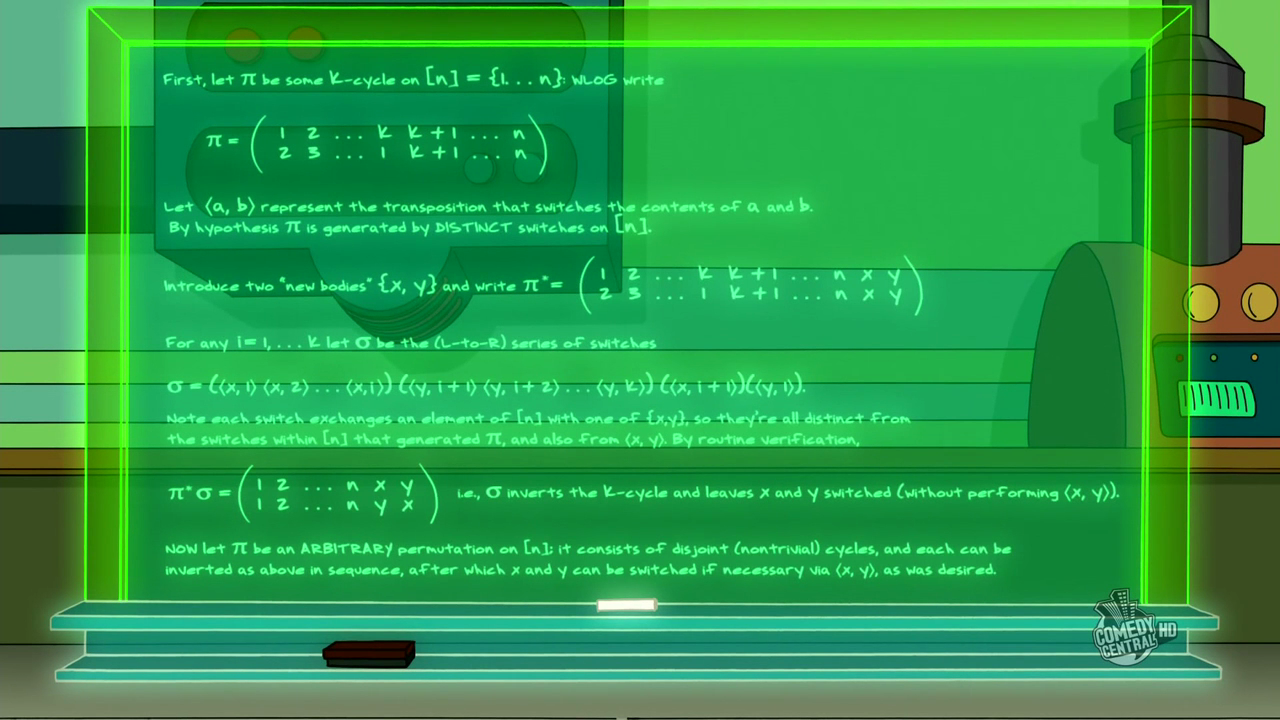Note:This image is taken from The Infosphere: The Futurama Wiki.

## Cycle Notation

Before describing the solution, we need to introduce a more efficient way of encoding permutations. One such method is called cycle notation.

### Example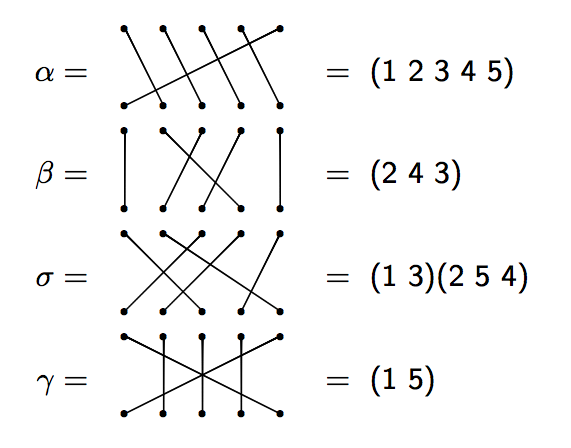## Cycle Notation

Let's try multiplying using cycle notation. Reminder: We multiply right to left (like function composition).

### Example

Consider $\alpha, \beta$, and $\gamma$ in $S_{5}$ as in the previous examples. Then

$\alpha \beta=(1\; 2\; 3\; 4\; 5)(2\; 4\; 3)=(1\; 2\; 5).$

and

$\gamma\beta =(2\; 4\; 3)(1\; 5)=(1\; 5)(2\; 4\; 3).$

We saw earlier that $\beta$ and $\gamma$ commute with each other, which is why it looks like nothing happened in the second example.

• In cycle notation, the Futurama permutation is $(8\; 9)(1\; 2\; 3\; 4\; 5\; 6\; 7)$.
• The interpretation of a cycle $(\cdots\; i\; j\; \cdots)$ is that the body numbered $i$ passes its mind to the body numbered $j$, etc.
• Transpositions are of the form $(i\; j)$, which means that $i$ and $j$ swap minds.
• "Disjoint" cycles (including transpositions) commute.
• If we can figure out how to "fix" one cycle at a time, then we can likely fix them all.

## Fixing a Single 7-cycle

Consider just the 7-cycle $(1\; 2\; 3\; 4\; 5\; 6\; 7)$. Introduce two new bodies that have not had their minds swapped with anyone else, say $x$ and $y$. To return all minds of 1-7 back to their rightful owner, multiply the cycle (on the left) by the following sequence of transpositions:

$(x\; 7)(y\; 1)(y\; 2)(y\; 3)(y\; 4)(y\; 5)(y\; 6)(y\; 7)(x\; 1)$

Let's verify that this actually works! First, notice that all of these transpositions are distinct and since $x$ and $y$ are new, we never used any of the above transpositions to obtain our original scrambling of minds.

$(x\; 7)(y\; 1)(y\; 2)(y\; 3)(y\; 4)(y\; 5)(y\; 6)(y\; 7)(x\; 1)(1\; 2\; 3\; 4\; 5\; 6\; 7)=???$

Note: $x$ and $y$ now have their minds swapped with each other, but they never sat in the machine at the same time!

## Fixing an Arbitrary $k$-cycle

Consider the $k$-cycle $(1\; 2\; \ldots\; k)$. How do we "fix" this cycle?

As before, introduce two new bodies that have not used the machine, say $x$ and $y$. To fix a $k$-cycle, multiply the cycle (on the left) by the following:

$(x\; k)(y\; 1)(y\; 2)\cdots (y\; k-1)(y\; k)(x\; 1)$

Why does this work?!

• First observation: $(y\; k)(x\; 1)(1\; 2\; \ldots\; k-1\; k)=(1\; 2\; \ldots\; k-1\; y\; k\; x)$.
• Then $(y\; k-1)$ puts the mind of $k-1$ back where it belongs.
• And then $(y\; k-2)$ puts the mind of $k-2$ back where it belongs.
• We continue this way and in the second to last step, $(y\; 1)$ puts the mind of 1 back where it belongs.
• And finally, we finish by swapping $x$ and $k$.
• We now have $x$ and $y$ with their minds swapped (but they never sat in the machine at the same time).

## Fixing Products of Disjoint Cycles

What do we do when we have multiple cycles to start with?

• Use $x$ and $y$ to fix each individual cycle.
• When doing this, $x$ and $y$ will sometimes have their minds swapped (but they never sat in machine at same time).
• If the number of disjoint cycles is even, then we are done after we fix each individual cycle.
• If the number of disjoint cycles is odd, then after fixing each individual cycle, finish by having $x$ and $y$ sit in the machine at the same time.

## Observations and Questions

• Fun Problem: Which permutations in $S_n$ can we obtain by multiplying distinct transpositions? That is, what configurations of mind swaps are possible with $n$ people?
• Regardless of what the answer to the previous problem is, notice that the Futurama Theorem doesn't care how the permutation arose.
• In general, if $\sigma\in S_n$ consisting of $r$ disjoint cycles, then Keeler's algorithm requires $n+2r+1$ or $n+2r$ moves depending on whether $r$ is odd or even, respectively.
• Can we do better (i.e., fewer moves to fix the cycles)?
• Applying our algorithm to the arrangement of minds in Futurama requires 13 moves.
• But since Fry and Zoidberg only swapped with each other and since there is only one other cycle, we could use Fry and Zoidberg as $x$ and $y$, respectively in our algorithm. This only requires 9 moves.
• Under what circumstances do we not need to introduce two "clean" people?
• Evans and Huang address questions related to optimality in Mind Switches in Futurama and Stargate (Mathematics Magazine 2014).

## Stargate

• Stargate is an adventure military science fiction franchise. The first film was released in October 1994.
• The movies and TV shoes focus on "stargates", which are circular devices that create wormholes, enabling instantaneous transportation to another stargate located many light years away from the starting point.
• Stargate SG-1 is a television show based on the 1994 film. The series ran from 1997 to 2007.
• "Holiday" is a 1999 episode from Season 2 of Stargate SG-1. In the episode, the character Ma'chello tricks Daniel into swapping minds with him. In an attempt to save Daniel, Jack and Teal'c accidentally swap minds.
• They then discover a limitation: the machine will not work more than once on the same pair of bodies. Physicist Samantha Carter saves the day by improvising a sequence of 4 switches that brings everyone back to normal.

## The Stargate Switch Problem

Here are the swaps that take place during the episode:

• Ma'chello $\leftrightarrow$ Daniel
• Jack $\leftrightarrow$ Teal'c

The question is:

Can the machine be used to put everyone's mind back in the proper body if we are not allowed to use the machine on the same pair of bodies more than once?

## The Generalized Stargate Switch

• Suppose we have $m$ pairs of bodies, where each pair swaps minds.
• Can the machine be used to put everyone's mind back in the proper body if we are not allowed to use the machine on the same pair of bodies more than once?
• This is a special case of the problem posed in Futurama. So, we know this is solvable.
• However, do we need to introduce outsiders to solve the problem? Also, what is the minimal number of "moves" required to put everyone's mind back where it belongs?
• In cycle notation, the original Stargate Switch permutation can be written as $\sigma=(1\; 2)(3\; 4),$ where 1 is Ma'chello, 2 is Daniel, 3 is Jack, and 4 is Teal'c.
• The Generalized Stargate Switch permutation is of the form $\sigma=(1\; 2)(3\; 4)\cdots (2m-1\; 2m).$
• To solve the problem, we must produce a permutation $\mu$ consisting of distinct transpositions, none equaling a factor of $\sigma$, such that the product $\mu\sigma$ is the identity in $S_{2m}$.

## Solution to Original Stargate Switch

The following permutation solves the original Stargate Switch Problem.

$\mu=(2\; 4)(1\; 3)(2\; 3)(1\; 4)$

Moreover, this solution is optimal in the sense that no solution with fewer than 4 moves will work.

### Proof

First, easy to check that $\mu\sigma$ yields the identity:

$\mu\sigma=(2\; 4)(1\; 3)(2\; 3)(1\; 4)\circ (1\; 2)(3\; 4)=\text{identity permutation}$

Why is $\mu$ optimal? Well, it's easy to check that 2 transpositions (not reusing the originals and only using $1,2,3,4$) won't work. The reason why $\mu$ cannot only have 3 cycles is because of a general fact that says that the identity permutation cannot be equal to a product of an odd number of transpositions.

## Solution to Generalized Stargate Switch

Evans and Huang also proved the following more general theorem.

### Theorem

Let $m>1$. Then the Generalized Stargate Switch permutation

$\sigma=(1\; 2)(3\; 4)\cdots (2m-1\; 2m)$

can be undone by a product $\mu$ of $N(m)$ distinct transpositions, where for $j=4i$

$\mu=\begin{cases}\tau \prod_{i=2}^{(m-1)/2} (j\; j+2)(j-1\; j+1)(j\; j+1)(j-1\; j+2), & \text{if }m\text{ is odd}\\ \prod_{i=1}^{m/2}(j-1\; j)(j-3\; j-1)(j-2\; j-1)(j-3\; j), & \text{if }m\text{ is even}\end{cases}$

with

$\tau=(1\; 3)(1\; 6)(4\; 5)(4\; 6)(3\; 5)(2\; 5)(1\; 5).$

and

$N(m)=\begin{cases}2m+1, & \text{if }m\text{ is odd (and greater than 1),}\\ 2m, & \text{if }m\text{ is even.}\end{cases}$

The empty product for $m=3$ is interpreted as the identity permutation. Moreover, $N(m)$ is optimal.

## Examples of Solutions

The previous formula looks pretty scary, but it's not too bad.

### Example

When $m=3$, we have

$\mu= \tau=(1\; 3)(1\; 6)(4\; 5)(4\; 6)(3\; 5)(2\; 5)(1\; 5),$

which consists of $N(3)=7$ transpositions.

When $m=4$, we have

$\mu=(2\; 4)(1\; 3)(2\; 3)(1\; 4)(6\; 8)(5\; 7)(6\; 7)(5\; 8),$

which consists of $N(4)=8$ transpositions.